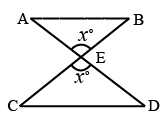# TS ICET 2015

Instructions

A question is followed by data in the form of two statements labelled as I and II. You must decide whether the data given in the statements are sufficient to answer the questions.

Question 1

# What are the numbers a and b ?I. a and b are relatively prime.II. L.C.M of a, b is 2550Question 2

# What is the rate of simple interest on a loan of ₹ 20,000 ?I. ₹ 23,000 is to be paid at the end of the first year.II. There is a penal interest of 10% if the loan is not paid at the end of the first year.Question 3

# Suppose f(x) is a polynomial. Is x - 1 a factor of $$f(x^2 + 7)$$?I. $$(x - 7) \mid f(x)$$II. $$(x - 11) \mid f(x - 3)$$Question 4

# What is the value of xy?I. $$x^2 = 9$$II. y = 0Question 5

# Are the triangles $$\triangle ABE$$ and $$\triangle CDE$$ given below congruent ?I. $$AB \parallel CD$$II. $$\angle AEB = \angle CED$$Question 6

# If $$0 \in \left\{x, y\right\}$$, then what is the value of x + y ?I. $$x \neq y$$II. $$x^8 + y = y^8 + x$$Question 7

# Is $$525 \times k$$ a perfect square?I. $$21 \mid k$$II. k = 21Question 8

# If n(A) denotes the number of elements in the set A, then what is $$n(A \cup B)$$ ?I. n(A) = 35II. n(B) = 28Question 9

# What is the value of the integer $$n \geq 0$$ ?I. $$25^n$$ is odd.II. $$30^n$$ is odd.Question 10

# For the integers a, b and c with a = bc, is a > b ?I. $$\mid a \mid = \mid b \mid$$II. $$c^2 = 9$$OR